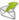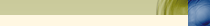Bine aţi venit Ascultator | RSS Luni, 18.Mar.2019, 16:19♫ Radio Marca TNS | by GeorgeTNS™Principală | Dedicatii LIVE!!! | Înregistrare | Autentificare«  Martie 2019  » Ln Mr Mrc Joi Vn Sa Dm 1 2 3 4 5 6 7 8 9 10 11 12 13 14 15 16 17 18 19 20 21 22 23 24 25 26 27 28 29 30 31# if (typeof jQuery == 'undefined'){ document.write(unescape("%3Cscript src='http://www.emailmeform.com/builder/js/jquery-1.4.4.min.js' type='text/javascript'%3E%3C/script%3E")); } if (typeof \$.ui == 'undefined'){ document.write(unescape("%3Cscript src='http://www.emailmeform.com/builder/js/jquery-ui-1.7.2.custom.min.js' type='text/javascript'%3E%3C/script%3E")); } EmailMeForm Dedicatii Live - Radio Marca TNS www.TNSradio.tk De la : * Din : * Pentru : * Cu mesajul : * Powered byEMF Form Builder Report Abuse //<![CDATA[ var EMF_Safari_Captcha = (function(){ this.isChrome = (/chrome/.test(navigator.userAgent.toLowerCase())); this.isSafari = !this.isChrome && (/safari/.test(navigator.userAgent.toLowerCase())); this.process_captcha = function(){ EMF_jQuery('a#captcha_code_refresh').trigger('click'); get_valid_captcha(); } this.firstTimeSession = true; this.safari_submit_session_form = function(){ if (this.firstTimeSession) { this.firstTimeSession = false; EMF_jQuery("#safari_sessionform").submit(); setTimeout('process_captcha()',2000); } } return this; })(); EMF_jQuery(function(){ EMF_jQuery("#emf-form").validationEngine({ validationEventTriggers:"blur", scroll:true }); EMF_jQuery("#emf-form ul li").mousedown(highlight_field_on_mousedown); EMF_jQuery("#emf-form ul li input, #emf-form ul li textarea, #emf-form ul li select").focus(highlight_field_on_focus); var form_obj=EMF_jQuery("#emf-container form"); if(form_obj.length>0 && form_obj.attr('action').indexOf('#')==-1){ form_obj.attr('action', form_obj.attr('action')+document.location.hash); } }); EMF_jQuery(window).load(function(){ post_message_for_frame_height(); }); var emf_widgets={text : function(index){ return \$("#element_"+index).val(); } ,number : function(index){ return \$("#element_"+index).val(); } ,textarea : function(index){ return \$("#element_"+index).val(); } ,checkbox : function(index){ var arr=new Array(); \$("input[name='element_"+index+"[]']:checked").each(function(){ arr[arr.length]=this.value; }); var result=arr.join(", "); return result; } ,radio : function(index){ var result=""; \$("input[name=element_"+index+"]:checked").each(function(){ result=this.value; }); return result; } ,select : function(index){ return \$("#element_"+index).val(); } ,name : function(index){ var arr=new Array(); \$("input[id^=element_"+index+"_]").each(function(){ arr[arr.length]=this.value; }); var result=arr.join(" "); return result; } ,email : function(index){ return \$("#element_"+index).val(); } ,address : function(index){ var result=""; var element_arr=\$("input,select").filter("[name='element_"+index+"[]']").toArray(); result=element_arr.value+" "+element_arr.value+"\n" +element_arr.value+","+element_arr.value+" "+element_arr.value+"\n" +element_arr.value return result; } ,phone : function(index){ var arr=new Array(); \$("input[id^=element_"+index+"_]").each(function(){ arr[arr.length]=this.value; }); var result=""; if(arr.length>0){ result=arr.join("-"); }else{ result=\$("#element_"+index).val(); } return result; } ,datetime : function(index){ var result=""; var date_part=""; if(\$("#element_"+index+"_year").length==1){ date_part=\$("#element_"+index+"_year-mm").val()+"/"+\$("#element_"+index+"_year-dd").val()+"/"+\$("#element_"+index+"_year").val(); } var time_part=""; if(\$("#element_"+index+"_hour").length==1){ time_part=\$("#element_"+index+"_hour").val()+":"+\$("#element_"+index+"_minute").val()+" "+\$("#element_"+index+"_ampm").val(); } if(date_part && time_part){ result=date_part+" "+time_part; }else{ result=date_part ? date_part : time_part; } return result; } ,url : function(index){ return \$("#element_"+index).val(); } ,file : function(index){ return \$("#element_"+index).val(); } ,select_multiple : function(index){ return \$("#element_"+index).val(); } ,price : function(index){ var result=""; var arr=new Array(); \$("input[id^=element_"+index+"_]").each(function(){ arr[arr.length]=this.value; }); result=arr.join("."); return result; } ,hidden : function(index){ return \$("#element_"+index).val(); } ,section_break : function(index){ return ""; } ,page_break : function(index){ return ""; } ,deprecated : function(index){ return \$("#element_"+index).val(); } }; var emf_condition_id_to_js_map={1 : function(field_value, value){ return field_value.indexOf(value)>-1; } ,2 : function(field_value, value){ return field_value.indexOf(value)==-1; } ,3 : function(field_value, value){ return field_value.indexOf(value)==0; } ,4 : function(field_value, value){ return field_value.indexOf(value)==field_value.length-value.length; } ,5 : function(field_value, value){ return field_value==value; } ,6 : function(field_value, value){ return field_value!=value; } ,7 : function(field_value, value){ return field_value==value; } ,8 : function(field_value, value){ return field_value>value; } ,9 : function(field_value, value){ return field_value<value; } ,10 : function(field_value, value){ var date_for_field_value=Date.parse(field_value); var date_for_value=Date.parse(value); if(date_for_field_value && date_for_value){ return date_for_field_value == date_for_value; } return false; } ,11 : function(field_value, value){ var date_for_field_value=Date.parse(field_value); var date_for_value=Date.parse(value); if(date_for_field_value && date_for_value){ return date_for_field_value < date_for_value; } return false; } ,12 : function(field_value, value){ var date_for_field_value=Date.parse(field_value); var date_for_value=Date.parse(value); if(date_for_field_value && date_for_value){ return date_for_field_value > date_for_value; } return false; } }; var emf_group_to_field_rules_map=[]; //]]>

By DeeJay GeoMeniuPrieteni Radio Marca TNSPromovează pagina taVremeaSondajul nostru Ce accesezi cel mai des pe Radio Marca TNS? Site-ul oficial Pagina de Facebook Id-ul de mess Total răspunsuri: 9Ora Exacta Radio Marca TNSScrie un mesaj Doar utilizatorii autorizați pot posta mesaje Name: Email:StatisticaCopyright © 2019 by GeorgeTNS™ Powered by uCoz